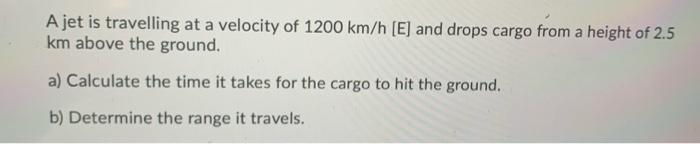# Question Solved1 AnswerA jet is travelling at a velocity of 1200 km/h (E) and drops cargo from a height of 2.5 km above the ground. a) Calculate the time it takes for the cargo to hit the ground. b) Determine the range it travels.Transcribed Image Text: A jet is travelling at a velocity of 1200 km/h (E) and drops cargo from a height of 2.5 km above the ground. a) Calculate the time it takes for the cargo to hit the ground. b) Determine the range it travels.
More
Transcribed Image Text: A jet is travelling at a velocity of 1200 km/h (E) and drops cargo from a height of 2.5 km above the ground. a) Calculate the time it takes for the cargo to hit the ground. b) Determine the range it travels.